# Научный форум dxdy

Математика, Физика, Computer Science, Machine Learning, LaTeX, Механика и Техника, Химия,
Биология и Медицина, Экономика и Финансовая Математика, Гуманитарные науки

 Печатать страницу | Печатать всю тему Пред. тема | След. тема

 Re: Al Zimmermann's: Statue of Liberty06/04/20
4
 porcoroso в сообщении #1452649 писал(а):So for 80^15 I get:80^15=>{74,73,70,69,67,66,64,62,54,53,52,51,50,49,47,45,44,39,37,36,33,30,29,28,26,22,20,18,17,16,14,13,12,10,8,7,5,4,3,2,1}Can anyone here verify that at least this data point is correct ?I get that too.Re: Al Zimmermann's: Statue of Liberty01/06/12
1016
 Последний раз редактировалось dimkadimon 08.04.2020, 10:58, всего редактировалось 5 раз(а). Well a friend of mine just found the optimal for ^16. First this gave me some hope. But then he said that it was a massive effort for him and he is one of the best marathoners at TopCoder, so all my hopes were completely destroyed...Speaking of TopCoder, I frequently write problems for their long competitions (1 week). They are really fun and competitive! So I invite you all to participate. The next one is on 22nd April.-- 08.04.2020, 16:26 --porcoroso в сообщении #1452649 писал(а):80^15=>{74,73,70,69,67,66,64,62,54,53,52,51,50,49,47,45,44,39,37,36,33,30,29,28,26,22,20,18,17,16,14,13,12,10,8,7,5,4,3,2,1}as optimal with minimal error 2917618Haha I did find this too, but it took me 15 minutes!-- 08.04.2020, 16:39 --So Fermat's last theorem states that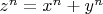$z^n = x^n + y^n$ has no solutions for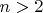$n>2$. But what do we know about a more generalised version that contains more terms. We know that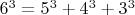$6^3 = 5^3 + 4^3 + 3^3$ and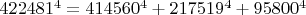$422481^4=414560^4+217519^4+95800^4$ (due to Roger Frye, 1988). But does$w^n = x^n + y^n + z^n$ have solutions for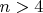$n>4$? What happens when we have more terms on the right hand side?Re: Al Zimmermann's: Statue of Liberty21/05/16
4294
Аделаида
 Последний раз редактировалось kotenok gav 08.04.2020, 11:46, всего редактировалось 1 раз. dimkadimon в сообщении #1452657 писал(а):What happens when we have more terms on the right hand side?It is a Euler equation, there is a hypothesis, that number of terms on one side plus power of equation is greater or equal than number of terms on other side.-- 08 апр 2020, 19:16 --For example, in this case we try to find solution to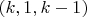$(k, 1, k-1)$.Re: Al Zimmermann's: Statue of Liberty20/01/13
62
 It is believed that all (k,1,k-1) have a solution.Currently, we have verified that relation for k<=5.In fact, I believe that (k,m,k-m) has always a solution, and this was verified for k<=8.About "IR", it's on my site euler.free.frWe found solutions like (32,16,158), in other words the sum of 16 32th powers is equal to the sum of 158 32th powers.Do you really believe that we were able to find such solutions via addition/subtraction?Re: Al Zimmermann's: Statue of Liberty01/06/12
1016
 Guys, IR = Integer Relation. But I am still not sure how to apply it to this problem.Re: Al Zimmermann's: Statue of Liberty20/01/13
62
 dimkadimon в сообщении #1452810 писал(а):Guys, IR = Integer Relation. But I am still not sure how to apply it to this problem.Indeed. In fact, there are 3 approaches on ESLP: - decomposing by computing with multiprecision (using modular constraints if possible)- decomposing by computing with modular residues (instead of doing multiprecision, we use prime numbers moduloes)- applying IR (there is a lot of research on this domain, because of cryptography)I'm not sure that it's the correct approach, but if done correctly, it should help solve all the large N.Re: Al Zimmermann's: Statue of Liberty07/06/16
45
Омск
 dimkadimon в сообщении #1452657 писал(а):porcoroso в сообщении #1452649 писал(а):80^15=>{74,73,70,69,67,66,64,62,54,53,52,51,50,49,47,45,44,39,37,36,33,30,29,28,26,22,20,18,17,16,14,13,12,10,8,7,5,4,3,2,1}as optimal with minimal error 2917618Haha I did find this too, but it took me 15 minutes!My program did it for 640 sec.I probably should be glad that my algorithm is not the most inhibitory. :-/Re: Al Zimmermann's: Statue of Liberty01/06/12
1016
 Jarek found ^26 and took the lead! Great competition.Re: Al Zimmermann's: Statue of Liberty25/08/12
171
Germany
 If the raw score is now 1-1/s for a perfect solution, we deduce from the best rawscores for k= 16, 17, 18, 19 and 20 for the corresponing s values220, 190, 234, 1061 and 5138.Very interesting.Re: Al Zimmermann's: Statue of Liberty06/04/20
4
 Its very interesting, but for me kind of disheartening, as I have no idea how to use modular constraints which now seems the only way to go, I am so bad at number theory. I was reading a lot lately about lattice techniques (LLL andother stuff) which is kind of relevant for general knapsack problems, but this ones seems out of reach.Jareks last solution seems to be with s > 2000,000 . Sigh ...Re: Al Zimmermann's: Statue of Liberty20/01/13
62
 porcoroso в сообщении #1453700 писал(а):Its very interesting, but for me kind of disheartening, as I have no idea how to use modular constraints which now seems the only way to go, I am so bad at number theory. I was reading a lot lately about lattice techniques (LLL andother stuff) which is kind of relevant for general knapsack problems, but this ones seems out of reach.Jareks last solution seems to be with s > 2000,000 . Sigh ...Modular constraints are quite easy to understand, once you play with the various modulo (especially 2^n).In my opinion, they are only interesting on 16th power, since you have a lot of restrictions.I believe there is a paper grom Giovanni Resta and myself that will explain how it works for 6th power, where you have the modular constraints of 2^6, 3^6 and 7^6.From what I remember on 16th power, if the left term is odd, then one of the right terms is odd, and most of the other right terms are even.If the left term is even, then:- either all the right terms are even (which makes the solution completely divisible by 2)- either 64 right terms are odd, and the other ones are even (I'm not sure about this one)And, despite my efforts, I was unable to get something relevant with LLL/BKZ yet.Re: Al Zimmermann's: Statue of Liberty07/06/16
45
Омск
 Последний раз редактировалось Archimedes 12.04.2020, 02:30, всего редактировалось 1 раз. Ну, я пока летаю низко.Переписал алгоритм на C#, используя простую арифметику и максимально простые структуры и алгоритмы. В общем, всё как говорили наши иностранные товарищи. :) Плюс включил в свойствах проекта оптимизацию под x64 (да, я как лох этого не делал раньше).В результате 80^15 выполняется за 126 сек. То есть, получил более чем 5-кратное ускорение.Плюс исчез memory-leak, который был при использовании объектов java.BigInteger. Да и вообще, программа стала использовать ОЗУ заметно меньше.Поэтому, если раньше на R5 2600 в ОЗУ помещалось только 5 экземпляров программы с данными, то сейчас, думаю, смогу использовать все 12 потоков.PS Видимо нужно объяснить почему C#. С того времени, когда я писал программы на С, используя ассемблерные вставки, слишком многое изменилось. Мне не удалось заставить ни одну из имеющихся у меня сред компилировать такой код для x64 и qword. Включая Visual Studio 2015, которой я пользуюсь. Поэтому, убив на разборки с компиляторами пару дней, я плюнул на всё и написал на том, чем владею в достаточной степени. Предполагаю что написанный оптимально код на C++/ASM дал бы те самые 30 секунд. Но пока на это нет времени. Полученный выигрыш в 5 раз - это уже хороший промежуточный результат. Надеюсь ещё получить некоторое ускорение оптимизациями кода и настроек.Re: Al Zimmermann's: Statue of Liberty25/08/12
171
GermanyRe: Al Zimmermann's: Statue of Liberty01/06/12
1016
 Последний раз редактировалось dimkadimon 13.04.2020, 16:03, всего редактировалось 1 раз. There are some interesting ways to relax the optimisation problem to make it easier:1. Allow coefficients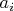$a_i$ to be greater than 1. So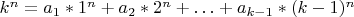$k^n = a_1*1^n+a_2*2^n + \ldots + a_{k-1}*(k-1)^n$. 2. Allow coefficients$a_i$ to be -1, 0 or +1 only. So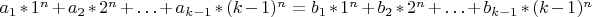$a_1*1^n+a_2*2^n + \ldots + a_{k-1}*(k-1)^n = b_1*1^n+b_2*2^n + \ldots + b_{k-1}*(k-1)^n$.As we force the coefficients to be 0 or 1 we go towards a solution for our problem. I have tried both approaches without much luck though.Re: Al Zimmermann's: Statue of Liberty25/08/12
171
Germany
 In Mathematica LatticeReduce[] implements the LLL algorithm. In the reduced lattice indeed we can search for entries with only -1,0, or 1. This works without problem for smaller n, for example n=8. Here with LatticeReduce we find for example immediately (using a 22x23 matrix)1^8 + 11^8 + 14^8 + 17^8 + 22^8 = 3^8 + 4^8 + 5^8 + 6^8 + 8^8 + 10^8 + 20^8 + 21^8 But this is no big help since for n>=16 all entries also contain larger coefficients.Показать сообщения за: Все сообщения1 день7 дней2 недели1 месяц3 месяца6 месяцев1 год Поле сортировки АвторВремя размещенияЗаголовок по возрастаниюпо убыванию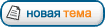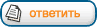Страница 4 из 7 [ Сообщений: 96 ] На страницу Пред.  1, 2, 3, 4, 5, 6, 7  След.

Модераторы: Karan, Toucan, PAV, maxal, Супермодераторы

#### Кто сейчас на конференции

Сейчас этот форум просматривают: нет зарегистрированных пользователей

 Вы не можете начинать темыВы не можете отвечать на сообщенияВы не можете редактировать свои сообщенияВы не можете удалять свои сообщенияВы не можете добавлять вложения

 Найти: